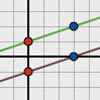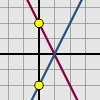#### You may also like### Parallel Lines

How does the position of the line affect the equation of the line? What can you say about the equations of parallel lines?### Diamond Collector

Collect as many diamonds as you can by drawing three straight lines.### Reflecting Lines

Investigate what happens to the equations of different lines when you reflect them in one of the axes. Try to predict what will happen. Explain your findings.

# Enclosing Squares

##### Age 11 to 14Challenge Level

Why do this problem?
This activity enables learners to explore the concept of gradient and it follows on nicely from Perpendicular Lines . The lengths of the sides of the square being equal connects with Pythagoras Theorem and the property that, for perpendicular lines the product of the gradients is -1 (reversing the horizontal and vertical increments).

Possible Approach
The problem can be used in the early stages of working on gradients and before introducing the equation of a straight line in 2D. At this stage it will be challenging for the majority of learners and take up most of a lesson.

Although it is tagged as Stage 3, the problem can also be used at Stage 4 or 5 when it could be completed in 10 to 15 minutes.

At Stage 5, extension work could ask for the equation of the circle through the 4 vertices linking Pythagoras Theorem, the midpoint of a line segment, the distance between 2 points with given coordinates and the equation of a circle.

Key Questions
If you know the coordinates of two points how much do you go up and down on the line through the points when you go across one unit?

How do you know if 2 line segments are parallel?

How do you know if 2 line segments are perpendicular?

If you know the coordinates of two points how do you find the distance between them?

Questions for the Stage 5 Extension:
How do you find the midpoint of a square?

What do you know about points on a circle in relation to the centre of the circle?